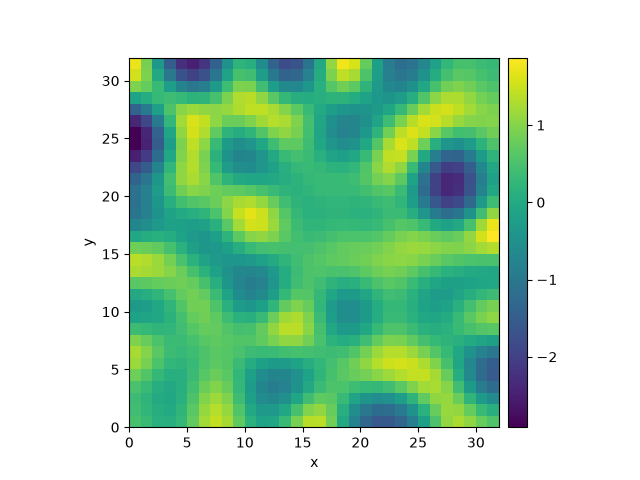# 2.6. Kuramoto-Sivashinsky - Using PDE class

This example implements a scalar PDE using the PDE. We here consider the Kuramoto–Sivashinsky equation, which for instance describes the dynamics of flame fronts:

$\partial_t u = -\frac12 |\nabla u|^2 - \nabla^2 u - \nabla^4 u$0%|          | 0/10.0 [00:00<?, ?it/s]
Initializing:   0%|          | 0/10.0 [00:00<?, ?it/s]
0%|          | 0/10.0 [00:15<?, ?it/s]
0%|          | 0.01/10.0 [00:27<7:44:49, 2791.70s/it]
0%|          | 0.02/10.0 [00:27<3:52:10, 1395.87s/it]
1%|          | 0.1/10.0 [00:27<46:03, 279.18s/it]
43%|████▎     | 4.33/10.0 [00:27<00:36,  6.45s/it]
43%|████▎     | 4.33/10.0 [00:27<00:36,  6.45s/it]
100%|██████████| 10.0/10.0 [00:27<00:00,  2.79s/it]
100%|██████████| 10.0/10.0 [00:27<00:00,  2.79s/it]


from pde import PDE, ScalarField, UnitGrid

grid = UnitGrid([32, 32])  # generate grid
state = ScalarField.random_uniform(grid)  # generate initial condition

eq = PDE({"u": "-gradient_squared(u) / 2 - laplace(u + laplace(u))"})  # define the pde
result = eq.solve(state, t_range=10, dt=0.01)
result.plot()


Total running time of the script: (0 minutes 28.157 seconds)